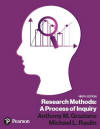﻿ Manual Computation of Statistics ExercisesGraziano & Raulin
Research Methods (9th edition)

## Practice with Manual Computations of Statistics

Listed below are several small data sets on which on can practice the manual computation of statistics. The computational procedures for these statistics range from relatively simple to exceedingly complex. We recommend that complex procedures be done with a statistical analysis computer program, such as SPSS for Windows.

1. Compute the mean of the following numbers: 48, 39, 44, 52, 66. (answer)
2. Compute the median for the above numbers. (answer)

3. Compute the variance and standard deviation for the above numbers. (answer)

4. If, instead of 66, the final score was 166, how would that change the mean and the median? (answer)

5. Compute the mean and standard deviation for each of the two variables listed below, as well as the correlation between the two variables. Is this correlation statistically significant? (answer)

 X Y 23 143 42 153 53 149 44 171 74 189 29 142 65 157 44 153 39 136
1. Compute an independent samples t-test for the data below. Also show the mean and standard deviation. (answer)

 Group 1 Group 2 11 17 16 27 25 25 23 28 26 20 19 20 18 23 22 14
1. Compute correlated-samples t-test for the following data. Report the means, standard deviations, correlation, and t-test. (answer)

 Condition 1 Condition 2 21 24 32 33 25 26 29 32 23 24 26 28 25 25
1. Computer a one-way ANOVA for the following data in which there are three independent groups of participants. Report the descriptive statistics by group and show the ANOVA summary table. (answer)

 Group 1 Group 2 Group 3 5 5 4 3 8 5 8 5 3 6 11 6 5 9 2 4 6 4 8 7 5 3 9 4 8 5 3 5 4 5